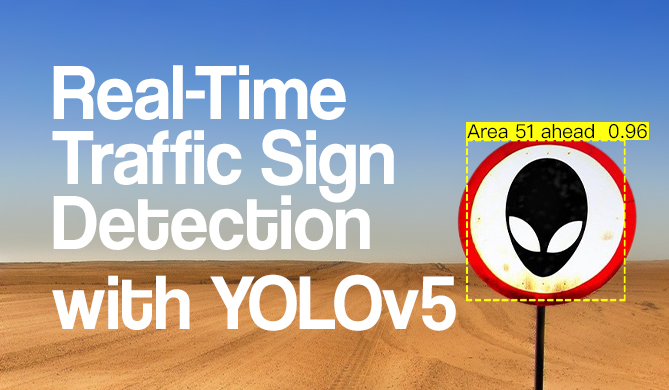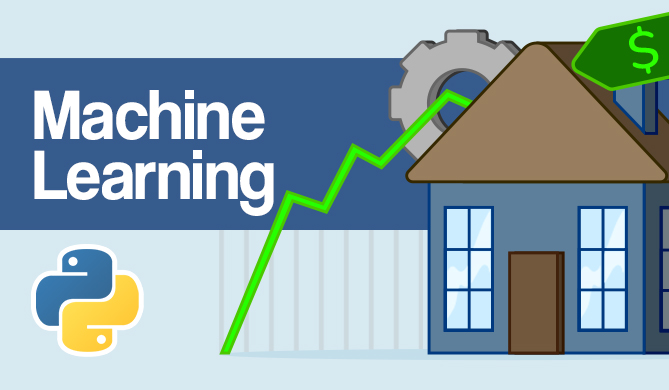End-to-End XGBoost Regression Pipeline with Scikit-Learn

# End-to-End XGBoost Regression Pipeline with Scikit-LearnRegression is a technique in statistics and machine learning, in which the value of an independent variable is predicted by its relationship with other variables.

Frameworks like Scikit-Learn and XGBoost make it easier than ever to perform regression with a wide variety of models - one of the recently well-adopted one being Gradient Boosting and XGBoost's variant, Extreme Gradient Boosting models.

XGBoost works well with Scikit-Learn, has a similar API, and can in most cases be used just like a Scikit-Learn model - so it's natural to be able to build pipelines with both libraries.

With Scikit-Learn pipelines, you can create an end-to-end pipeline in as little as 4 lines of code: load a dataset, perform feature scaling, and then feed the data into a regression model:

from sklearn import datasets
from sklearn.model_selection import train_test_split
from sklearn.preprocessing import MinMaxScaler
from sklearn.pipeline import Pipeline

from xgboost import XGBRegressor

X, y = datasets.fetch_california_housing(return_X_y=True)
X_train, X_test, y_train, y_test = train_test_split(X, y)

pipeline = Pipeline([('scaler', MinMaxScaler()), ('regressor', XGBRegressor())])
pipeline.fit(X_train, y_train)

r2 = pipeline.score(X_test, y_test)
print(f"XGBoost regressor r2: {r2}")
# XGBoost regressor r2: 0.8323581845230325


Alternatively, you can separate the steps outside of the pipeline, which is a bit more verbose, yet more flexible:

X, y = datasets.fetch_california_housing(return_X_y=True)
X_train, X_test, y_train, y_test = train_test_split(X, y)

scaler = MinMaxScaler()
X_train_scaled = scaler.fit_transform(X_train)
X_test_scaled = scaler.transform(X_test)

xbg_reg = XGBRegressor().fit(X_train_scaled, y_train)

r2 = xbg_reg.score(X_test_scaled, y_test)
print(f"XGBoost Regressor: {r2}")

# XGBoost regressor r2: 0.8294001231626023

Last Updated: July 1st, 2022

### You might also like...

David LandupAuthor

Entrepreneur, Software and Machine Learning Engineer, with a deep fascination towards the application of Computation and Deep Learning in Life Sciences (Bioinformatics, Drug Discovery, Genomics), Neuroscience (Computational Neuroscience), robotics and BCIs.

Great passion for accessible education and promotion of reason, science, humanism, and progress.Project

### Real-Time Road Sign Detection with YOLOv5

# python# machine learning# computer vision# pytorch

If you drive - there's a chance you enjoy cruising down the road. A responsible driver pays attention to the road signs, and adjusts their...David Landup
DetailsProject

### Hands-On House Price Prediction - Machine Learning in Python

# python# machine learning# scikit-learn# tensorflow

If you've gone through the experience of moving to a new house or apartment - you probably remember the stressful experience of choosing a property,...Details